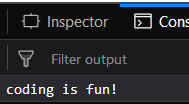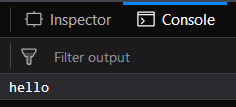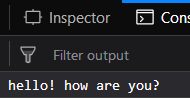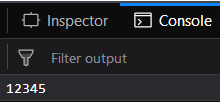Related Articles

# Underscore.js | _.delay() with Examples

• Last Updated : 03 May, 2019

_.delay() function:

• It executes the mentioned function in its argument after waiting for the specified milliseconds.
• It is mostly used when we want to perform some task but after a certain amount of time. In this case we can define this function and then it will be executed after the wait milliseconds.
• If we pass the arguments also to this function (which is optional to pass) then these arguments will act as the argument of the function passed to the _.delay() function.

Syntax:

Hey geek! The constant emerging technologies in the world of web development always keeps the excitement for this subject through the roof. But before you tackle the big projects, we suggest you start by learning the basics. Kickstart your web development journey by learning JS concepts with our JavaScript Course. Now at it's lowest price ever!

`_.delay(function, wait, *arguments)`

Parameters: It takes three arguments:

• The function to be executed.
• wait: The time after which the function needs to be executed ( in millisecond)
• The argument for the function passed to the _.delay() function (optional)

Return value: It returns the values of the function passed, being executed after wait millisecond.

Examples:

1. Passing the function directly to the _.delay() function: The _.delay() function takes the wait parameter which here is 1000ms, then waits for 1000ms and then executes the function passed which here is console.log() and prints the string passed to it, i.e., coding is fun. So, after 1000ms the string “coding is fun” will be displayed.
 `<``html``>``  ` `<``head``>``    ````    ``<``meta` `content``=``"text/html;charset=utf-8"` `http-equiv``=``"Content-Type"``>``    ``<``meta` `content``=``"utf-8"` `http-equiv``=``"encoding"``>``    ````    ``<``script` `src` `= ``"https://cdnjs.cloudflare.com/ajax/libs/underscore.js/1.9.1/underscore-min.js"``>``    ``````  ` `<``body``>``    ``<``script` `type``=``"text/javascript"``>``        ``_.delay(console.log, 1000, 'coding is fun!');``    ``````  ` ``

Output:2. Using _.bind() Function with the _.delay() function:
_.bind() function is used to pass the object to the function. Like here the console.log() function has an object console. This ‘func()’ means that whatever will be passed to this function will be displayed on the console. No waiting time is mentioned in the _.bind() function. Then in the _.delay() function we need to wait 2000 msec and after that the string “hello” will be displayed onto the console.
 `<``html``>``  ` `<``head``>``    ````    ``<``meta` `content``=``"text/html;charset=utf-8"` `http-equiv``=``"Content-Type"``>``    ``<``meta` `content``=``"utf-8"` `http-equiv``=``"encoding"``>``    ````    ``<``script` `src` `= ``"https://cdnjs.cloudflare.com/ajax/libs/underscore.js/1.9.1/underscore-min.js"` `>``    ````    ``<``script` `src``=``    ``"https://ajax.googleapis.com/ajax/libs/jquery/3.3.1/jquery.min.js"``>``    ``````  ` `<``body``>``    ``<``script` `type``=``"text/javascript"``>``        ``var func = _.bind(console.log, console);``        ``_.delay(func, 2000, 'hello');``    ``````  ` ``

Output:3. Passing more than just one argument to the function passed to _.delay() function:
The _.delay() function has a ‘func()’ passed to it which contains the same _.bind() function as in the earlier example. Then a waiting time of 3000 msec is passed which means output will be displayed after 3000 msec. There are 3 more parameters passed which will be considered as the parameters of the ‘func()’ function passed. Therefore, the final output will be displayed after 3000 msec and will be a combination of all the 3 string, i.e., “hello! how are you?”.
 `<``html``>``  ` `<``head``>``    ````    ``<``meta` `content``=``"text/html;charset=utf-8"` `http-equiv``=``"Content-Type"``>``    ``<``meta` `content``=``"utf-8"` `http-equiv``=``"encoding"``>`` ` `    ````    ``<``script` `src` `= ``"https://cdnjs.cloudflare.com/ajax/libs/underscore.js/1.9.1/underscore-min.js"``>``    ````    ``<``script` `src``=``    ``"https://ajax.googleapis.com/ajax/libs/jquery/3.3.1/jquery.min.js"``>``    ``````  ` `<``body``>``    ``<``script` `type``=``"text/javascript"``>``        ``var func = _.bind(console.log, console);``        ``_.delay(func, 3000, 'hello!', 'how are', 'you?');``    ``````  ` ``

Output:4. Passing a number as the parameter to the passed function to the _.delay() function:
We can even pass numbers as argument tot he passed function. Here, we are passing ‘12345’ as the argument to the ‘func()’ function. The ‘func()’ function is declared as it is in earlier examples. The output of this function will be “12345” which will be displayed after 5000msec.
 `<``html``>``  ` `<``head``>``    ````    ``<``meta` `content``=``"text/html;charset=utf-8"` `     ``http-equiv``=``"Content-Type"``>``    ``<``meta` `content``=``"utf-8"` `http-equiv``=``"encoding"``>`` ` `    ````    ``<``script` `src` `= ``"https://cdnjs.cloudflare.com/ajax/libs/underscore.js/1.9.1/underscore-min.js"``>``    ````    ``<``script` `src``=``    ``"https://ajax.googleapis.com/ajax/libs/jquery/3.3.1/jquery.min.js"``>``    ``````  ` `<``body``>``    ``<``script` `type``=``"text/javascript"``>``        ``var func = _.bind(console.log, console);``        ``_.delay(func, 5000, '12345');``    ``````  ` ``

Output:NOTE: These commands will not work in Google console or in firefox as for these additional files need to be added which they didn’t have added.
 ```<``script` `type``=``"text/javascript"` `src` `=``"https://cdnjs.cloudflare.com/ajax/libs/underscore.js/1.9.1/underscore-min.js"``>```